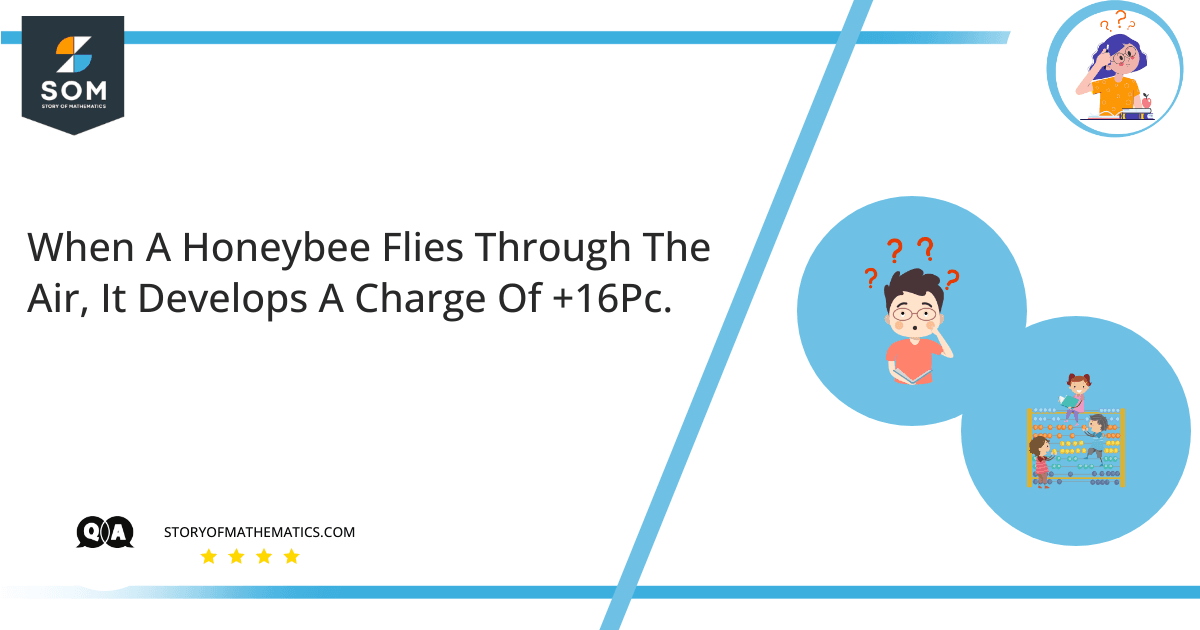# When a honeybee flies through the air, it develops a charge of +16pC.Calculate the number of electrons the honeybee loses while it develops the given charge while it flies.

The aim of this article is to find the number of electrons being lost by the honeybee while it acquires a positive charge of +16pC as it flies through the air.

Electric charge is the charge possessed by subatomic particles like protons, electrons, and neutrons. Protons caries positive electric charge whereas negative electric charge is carried by electrons. Neutrons are neutral and do not carry any electric charge.

Electric Charge is represented by the symbol $Q$ or $q$ and the total electric charge that is present in a body is equal to the number of electrons that the body carries multiplied by the standard electric charge of an electron as represented by the following formula:

$Q\ =\ n\ . e$

Where:

Q = Electric charge on the body

n = Number of electrons

e = Electric Charge on an electron

The SI unit for Electric Charge acquired by a body is Coulomb, which is represented by C.

As a standard, the electric charge on an electron is $1.6\times{10}^{-19}$

Given that:

Electric Charge on the Honeybee $Q\ =\ +16pC\ =\ +16\times{10}^{-12}\ C$

The number of Electrons $n=?$

When a honeybee flies, it has acquired a positive charge but at the same time, it loses a negative charge in terms of an electron as per the principles of conservation of electric charge which states that an electric charge can neither be created nor be destroyed but it is transferred from one system to the other. So the net total charge of that system remains the same.

We know that the total charge that has been developed by the honeybee can be represented as follows

$Q=n\ . e$

By substituting the values of $Q$ and $e$ in the above expression, we get:

$16\ \times\ {10}^{-12}\ C\ =\ n\ \times\ (1.6\ \times\ {10}^{-19}\ C)$

By rearranging the equation:

$n\ =\ \frac{16\ \times\ {10}^{-12}\ C\ }{1.6\ \times\ {10}^{-19}\ C}$

$n\ =\ 10\ \times\ {10}^{-12}\ \times\ {10}^{19}$

$n\ =\ 10\ \times\ {10}^{-12}\ \times\ {10}^{19}$

$n\ =\ {10}^8$

The number of Electrons is $n\ =\ {10}^8$

## Numerical Result

The number of electrons the honeybee loses while it develops the given charge while it flies is as follows:

$n\ =\ {10}^8$

## Example

When a plastic ball is thrown in the air it develops a charge of +20pC. Calculate the number of electrons the plastic ball loses while it develops the given charge while it moves in the air.

Given that:

Electric Charge on the Plastic Ball $Q\ =\ +\ 20\ pC\ =\ +\ 20\ \times\ {10}^{-12}\ C$

As we know:

$Q=n\ . e$

So:

$20\ \times\ {10}^{-12}\ C\ =\ n\ \times\ (1.6\ \times\ {10}^{-19}\ C)$

$n\ =\ \frac{20\ \times\ {10}^{-12}\ C\ }{1.6\ \times\ {10}^{-19}\ C}$

$n\ =\ 12.5\times{10}^7$

The number of electrons lost by Plastic Ball is:

$n\ =\ 12.5\times{10}^7$